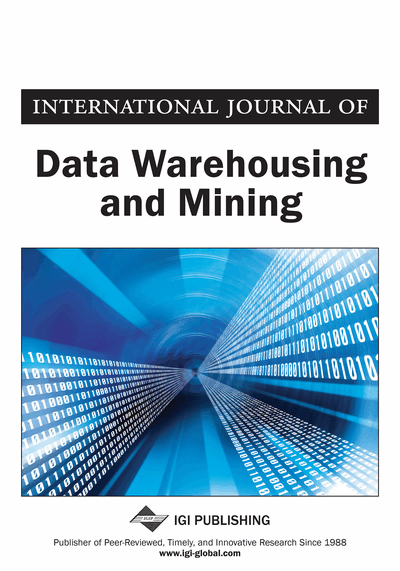# An Association Rules Based Approach to Predict Semantic Land Use Evolution in the French City of Saint-Denis

Asma Gharbi (LIASD Lab, University of Paris 8, Saint-Denis, France), Cyril de Runz (CReSTIC, University of Reims Champagne-Ardenne, Reims, France), Sami Faiz (Department of Computer Science, ISAMM, University of La Manouba, Tunisia) and Herman Akdag (LIASD Lab, University of Paris 8, Saint Denis, France)
DOI: 10.4018/ijdwm.2014040101

## Abstract

This paper proposes a predictive approach of semantic land use changes based on data mining techniques, specifically association rules (AR). Its main idea is centered around studying the past to predict the future. In other words, applying association rules technique to discover rules governing the city's past land use changes, then use them to forecast future changes. Taking La Plaine Zone in the city of Saint-Denis as a study case, the authors applied the proposed approach, in the framework of a developed Qgis plugin (Predict). The results are then highlighted in a cartographic format. To assess the quality of semantic land use changes prediction using our predictive approach, the authors proposed the Prediction precision degree (P) as an evaluation metric. A resulting value of 66% is considered promising since in this case, the authors only relay on history of land use changes for the prediction process, while considering some other evolution factors could remarkably enhance the results.
Article Preview
Top

## Prediction Methods

Several studies addressed the urban evolution prediction and simulation, and made use of different techniques, in this respect. By investigating the literature, we noticed two major categories: the statistical variable based techniques and the rule based techniques.

Variable based techniques such as logistic regression and Markov Chains based models, handle mathematical and statistical urban variables, such as the extent value of built up areas, population density, distance to main roads, etc., and their interrelation to understand the urban reality then predict it by means of statistical and mathematical operations. Many predictive variable based models employed logistic regression, a type of regression analysis used for predicting the outcome of a categorial criterion variables, based on one or more predictor variables, for empirically forecasting the probabilities of events such as urban changes (Fang, Gertner, Sun, & Anderson, 2005).

In line with logistic regression based methods, Markov chain models were commonly used in the urban development prediction and simulation. A Markov chain based method is defined as a set of states and a set of transitions with associated probabilities, where the transitions emerging from a given state define a distribution over the possible next states. The homogeneous Markov chain is a special class of Markov chains that is widely applied in practical simulation problems. As an example of works proposing Markov chains based predictive models, we note the work of Bhagawat (2011) wherein he focused on the analysis of urban growth trends, examined the land use and land cover changes then used Markov chains models to predict the urban growth patterns. Markov chains based models have been applied mainly on vegetation types (Balzter, 2000) and recently on urban and non urban coverage changes (Lopez, Bocco, Mendoza, & Duhau, 2001; Weng, 2002).

## Complete Article List

Search this Journal:
Reset
Open Access Articles
Volume 16: 4 Issues (2020): 1 Released, 3 Forthcoming
Volume 15: 4 Issues (2019)
Volume 14: 4 Issues (2018)
Volume 13: 4 Issues (2017)
Volume 12: 4 Issues (2016)
Volume 11: 4 Issues (2015)
Volume 10: 4 Issues (2014)
Volume 9: 4 Issues (2013)
Volume 8: 4 Issues (2012)
Volume 7: 4 Issues (2011)
Volume 6: 4 Issues (2010)
Volume 5: 4 Issues (2009)
Volume 4: 4 Issues (2008)
Volume 3: 4 Issues (2007)
Volume 2: 4 Issues (2006)
Volume 1: 4 Issues (2005)
View Complete Journal Contents Listing